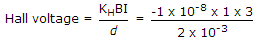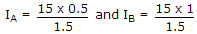# Electronics and Communication Engineering - Measurements and Instrumentation

### Exercise :: Measurements and Instrumentation - Section 3

1.

The current in a circuit is measured using a 150 : 1 CT If the ammeter reads 0.6 A, the circuit current is

 A. 250 A B. 90 A C. 156 A D. 144 A

Explanation:

150 x 0.6 = 90 A.

2.

A volt ratio box should have low resistance.

 A. True B. False

Explanation:

It should have high resistance.

3.

A Hall effect transducer has KH = - 1 x 10-8. If B = 1 Wb/m2, I = 3 A and bismuth slab is 2 mm wide, the Hall voltage is

 A. -7.5 x 10-6 V B. -15 x 10-6 C. -20 x 10-4 V D. -22.5 x 10-4 V

Explanation:.

4.

Two ammeters A and B both 0-10 A have internal resistance of 1 Ω and 0.5 Ω respectively. They are connected in parallel. If total current is 15 A, then

 A. IA = IB = 7.5 A B. IA = 5 A, IB = 10 A C. IA = 10 A, IB = 5 A D. IA = 9 A, IB = 6 A

Explanation:

Currents divide in inverse proportion of resistances..

5.

Which of the following is not correct?

 A. Voltmeter should have a very high resistance B. An ammeter should have a very low resistance C. A shunt should have a very low resistance D. An electronic voltmeter draws appreciable current from source